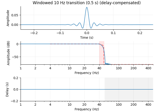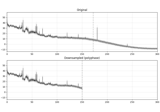# mne.filter.create_filter#

mne.filter.create_filter(data, sfreq, l_freq, h_freq, filter_length='auto', l_trans_bandwidth='auto', h_trans_bandwidth='auto', method='fir', iir_params=None, phase='zero', fir_window='hamming', fir_design='firwin', verbose=None)[source]#

Create a FIR or IIR filter.

`l_freq` and `h_freq` are the frequencies below which and above which, respectively, to filter out of the data. Thus the uses are:

• `l_freq < h_freq`: band-pass filter

• `l_freq > h_freq`: band-stop filter

• `l_freq is not None and h_freq is None`: high-pass filter

• `l_freq is None and h_freq is not None`: low-pass filter

Parameters:
data`ndarray`, shape (…, n_times) | `None`

The data that will be filtered. This is used for sanity checking only. If None, no sanity checking related to the length of the signal relative to the filter order will be performed.

sfreq`float`

The sample frequency in Hz.

l_freq

For FIR filters, the lower pass-band edge; for IIR filters, the lower cutoff frequency. If None the data are only low-passed.

h_freq

For FIR filters, the upper pass-band edge; for IIR filters, the upper cutoff frequency. If None the data are only high-passed.

filter_length

Length of the FIR filter to use (if applicable):

• ‘auto’ (default): The filter length is chosen based on the size of the transition regions (6.6 times the reciprocal of the shortest transition band for fir_window=’hamming’ and fir_design=”firwin2”, and half that for “firwin”).

• str: A human-readable time in units of “s” or “ms” (e.g., “10s” or “5500ms”) will be converted to that number of samples if `phase="zero"`, or the shortest power-of-two length at least that duration for `phase="zero-double"`.

• int: Specified length in samples. For fir_design=”firwin”, this should not be used.

l_trans_bandwidth

Width of the transition band at the low cut-off frequency in Hz (high pass or cutoff 1 in bandpass). Can be “auto” (default) to use a multiple of `l_freq`:

```min(max(l_freq * 0.25, 2), l_freq)
```

Only used for `method='fir'`.

h_trans_bandwidth

Width of the transition band at the high cut-off frequency in Hz (low pass or cutoff 2 in bandpass). Can be “auto” (default in 0.14) to use a multiple of `h_freq`:

```min(max(h_freq * 0.25, 2.), info['sfreq'] / 2. - h_freq)
```

Only used for `method='fir'`.

method`str`

‘fir’ will use overlap-add FIR filtering, ‘iir’ will use IIR forward-backward filtering (via filtfilt).

iir_params

Dictionary of parameters to use for IIR filtering. If iir_params is None and method=”iir”, 4th order Butterworth will be used. For more information, see `mne.filter.construct_iir_filter()`.

phase`str`

Phase of the filter. When `method='fir'`, symmetric linear-phase FIR filters are constructed, and if `phase='zero'` (default), the delay of this filter is compensated for, making it non-causal. If `phase='zero-double'`, then this filter is applied twice, once forward, and once backward (also making it non-causal). If `'minimum'`, then a minimum-phase filter will be constructed and applied, which is causal but has weaker stop-band suppression. When `method='iir'`, `phase='zero'` (default) or `phase='zero-double'` constructs and applies IIR filter twice, once forward, and once backward (making it non-causal) using filtfilt. If `phase='forward'`, it constructs and applies forward IIR filter using lfilter.

New in version 0.13.

fir_window`str`

The window to use in FIR design, can be “hamming” (default), “hann” (default in 0.13), or “blackman”.

New in version 0.15.

fir_design`str`

Can be “firwin” (default) to use `scipy.signal.firwin()`, or “firwin2” to use `scipy.signal.firwin2()`. “firwin” uses a time-domain design technique that generally gives improved attenuation using fewer samples than “firwin2”.

New in version 0.15.

verbose

Control verbosity of the logging output. If `None`, use the default verbosity level. See the logging documentation and `mne.verbose()` for details. Should only be passed as a keyword argument.

Returns:
filt

Will be an array of FIR coefficients for method=’fir’, and dict with IIR parameters for method=’iir’.

Notes

Note

For FIR filters, the cutoff frequency, i.e. the -6 dB point, is in the middle of the transition band (when using phase=’zero’ and fir_design=’firwin’). For IIR filters, the cutoff frequency is given by `l_freq` or `h_freq` directly, and `l_trans_bandwidth` and `h_trans_bandwidth` are ignored.

Band-pass filter

The frequency response is (approximately) given by:

```  1-|               ----------
|             /|         | \
|H| |            / |         |  \
|           /  |         |   \
|          /   |         |    \
0-|----------    |         |     --------------
|         |    |         |     |            |
0        Fs1  Fp1       Fp2   Fs2          Nyq
```

Where:

• Fs1 = Fp1 - l_trans_bandwidth in Hz

• Fs2 = Fp2 + h_trans_bandwidth in Hz

Band-stop filter

The frequency response is (approximately) given by:

```  1-|---------                   ----------
|         \                 /
|H| |          \               /
|           \             /
|            \           /
0-|             -----------
|        |    |         |    |        |
0       Fp1  Fs1       Fs2  Fp2      Nyq
```

Where `Fs1 = Fp1 + l_trans_bandwidth` and `Fs2 = Fp2 - h_trans_bandwidth`.

Multiple stop bands can be specified using arrays.

Low-pass filter

The frequency response is (approximately) given by:

```  1-|------------------------
|                        \
|H| |                         \
|                          \
|                           \
0-|                            ----------------
|                       |    |              |
0                      Fp  Fstop           Nyq
```

Where `Fstop = Fp + trans_bandwidth`.

High-pass filter

The frequency response is (approximately) given by:

```  1-|             -----------------------
|            /
|H| |           /
|          /
|         /
0-|---------
|        |    |                     |
0      Fstop  Fp                   Nyq
```

Where `Fstop = Fp - trans_bandwidth`.

New in version 0.14.

## Examples using `mne.filter.create_filter`#Background information on filtering

Background information on filteringFiltering and resampling data

Filtering and resampling data